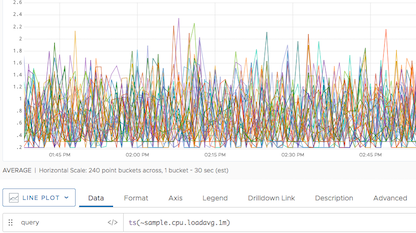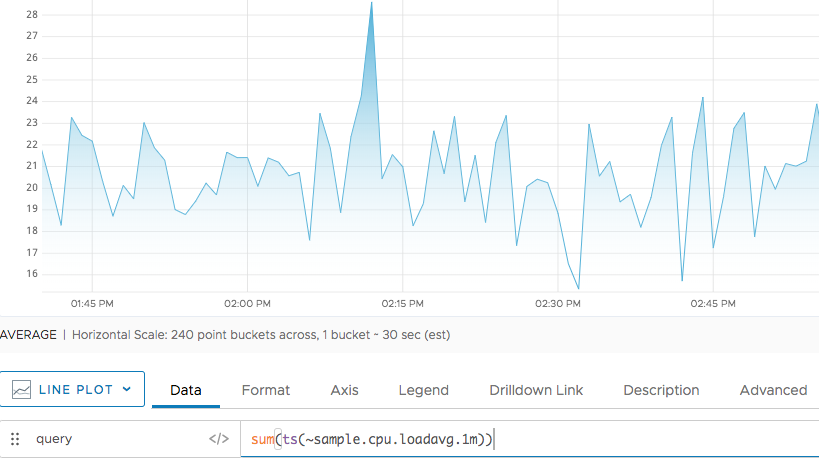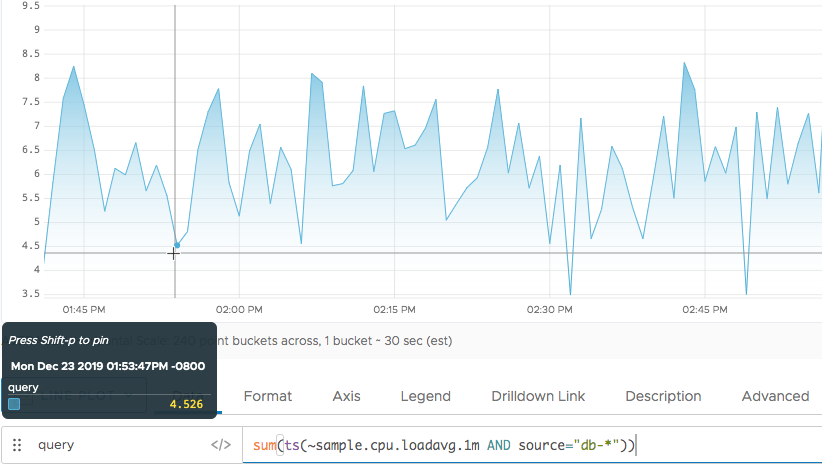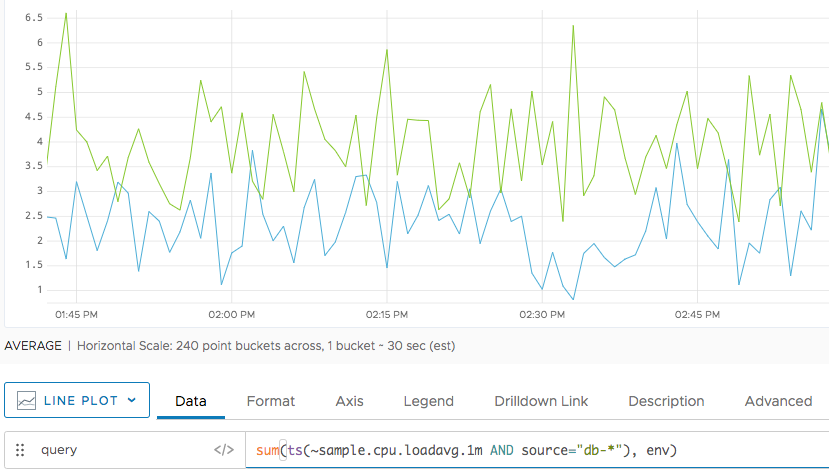Reference to the sum() function

## Summary

``````sum(<tsExpression>[,metrics|sources|sourceTags|pointTags|<pointTagKey>])
``````

Returns the sum of the set of time series described by the expression. The results might be computed from real reported values and interpolated values. Use `rawsum()` if you don’t need interpolation.

## Parameters

ParameterDescription
tsExpression Expression describing the set of time series to be summed.
metrics|sources|sourceTags|pointTags|<pointTagKey> Optional 'group by' parameter for organizing the time series into subgroups and then returning a sum for each subgroup.
• You can group using the literals metrics, sources, sourceTag, and pointTags to group by metric names, source names, source tag names, or point tag names.
• You can specify the name of a point tag key. For example, assume you have an env point tag with 2 possible values (prod and dev). If use an aggregation function and you group by env -- the point tag key -- we return one time series for env=prod and one for env=dev.
• You can combine multiple group by parameters.

## Description

The `sum()` aggregation function adds together the data values at each moment in time, across the time series that are represented by the expression.

By default, `sum()` produces a single series of sums by aggregating values across all time series. You can optionally group the time series based on one or more characteristics, and obtain a separate series of sums for each group.

If any time series has data gaps, `sum()` fills them in by interpolation whenever possible.

### Grouping

Like all aggregation functions, `sum()` returns a single series of results by default. You can include a ‘group by’ parameter to obtain separate subtotals for groups of time series that share common metric names, source names, source tags, point tags, or values for a particular point tag key. The function returns a separate series of results corresponding to each group.

You can specify multiple ‘group by’ parameters to group the time series based on multiple characteristics. For example, `sum(ts("cpu.cpu*"), metrics, Customer)` first groups by metric names, and then groups by the values of the `Customer` point tag.

### Interpolation

If any time series has gaps in its data, the query engine attempts to fill these gaps with interpolated values before applying the function. A value can be interpolated into a time series only if at least one other time series reports a real data value at the same moment in time.

Within a given time series, an interpolated value is calculated from two real reported values on either side of it. Sometimes interpolation is not possible–for example, when a new value has not been reported yet in a live-view chart. In this case, the query engine finds the last known reported value in the series, and assigns it to any subsequent moment in time for which a real reported data value is present in some other time series. We use the last known reported value only if interpolation can’t occur and if the last known reported value has been reported within the last 15% of the query time in the chart window.

You can use `rawsum()` to suppress interpolation. See Standard Versus Raw Aggregation Functions.

## Examples

The following set of examples uses the metrics returned by `ts(~sample.cpu.loadavg.1m)`. Each time series that’s returned reports CPU load averages from a particular source associated with particular point tag values. The following examples sum these series in different ways.Example 1: Use Sum with No Filtering, Noo Grouping

Here we include all of the time series in the results:

`sum(ts(~sample.cpu.loadavg.1m))`

Summing these series adds their values “vertically” to produce a single series of sums, so the chart shows a single line. For example, the aggregated value at around 2pm is the sum of the individual values reported by each time series at that time.Example 2: Filtering by Source

Here we filter the time series to include only those reported from `db-*` servers in the results. We still see a single line, but a different pattern. Notice that the aggregated value at changes because it’s the sum of values from fewer series.

`sum(ts(~sample.cpu.loadavg.1m AND source="db-*"))`Example 3: Grouping by Point Tag

Here we not only filter by source, but we also group the results to obtain separate subtotals for each `env` point tag value. This chart displays one line that sums the series with `env=production`, and a second line that sums the series with `env=dev`. This chart allows you to compare the CPU load patterns across the two groups of servers.

`sum(ts(~sample.cpu.loadavg.1m AND source="db-*") ,env)`## Caveats

Using `rawsum()` instead of `sum()` can significantly improve query performance because `rawsum()` does not perform interpolation.